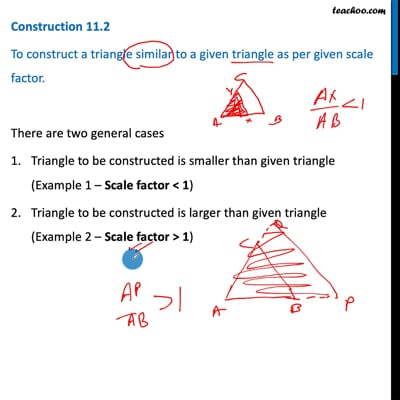Examples

Chapter 11 Class 10 Constructions [Deleted]
Serial order wise

Construction 11.2

To construct a triangle similar to a given triangle as per given scale factor.

There are two general cases

1. Triangle to be constructed is smaller than given triangle ( Example 1 Scale factor < 1 )
2. Triangle to be constructed is larger than given triangle ( Example 2 Scale factor > 1 )

Scale Factor means ratio of the sides of the triangle to be constructed with the corresponding sides of the given triangle

Scale Factor = (Side of to be constructed triangle)/(Side of given triangle)This video is only available for Teachoo black users

Maths Crash Course - Live lectures + all videos + Real time Doubt solving!

### Transcript

Construction 11.2 To construct a triangle similar to a given triangle as per given scale factor. There are two general cases Triangle to be constructed is smaller than given triangle (Example 1 – Scale factor < 1) Triangle to be constructed is larger than given triangle (Example 2 – Scale factor > 1) Scale Factor means ratio of the sides of the triangle to be constructed with the corresponding sides of the given triangle Scale Factor = (𝑆𝑖𝑑𝑒 𝑜𝑓 𝑡𝑜 𝑏𝑒 𝑐𝑜𝑛𝑠𝑡𝑟𝑢𝑐𝑡𝑒𝑑 𝑡𝑟𝑖𝑎𝑛𝑔𝑙𝑒)/(𝑆𝑖𝑑𝑒 𝑜𝑓 𝑔𝑖𝑣𝑒𝑛 𝑡𝑟𝑖𝑎𝑛𝑔𝑙𝑒)